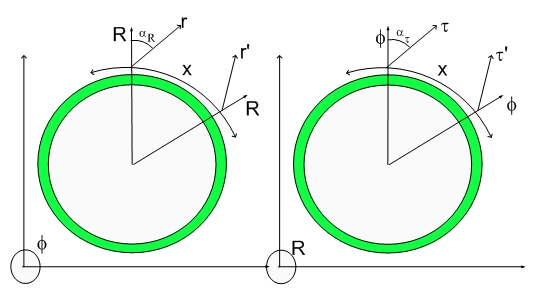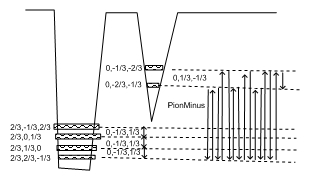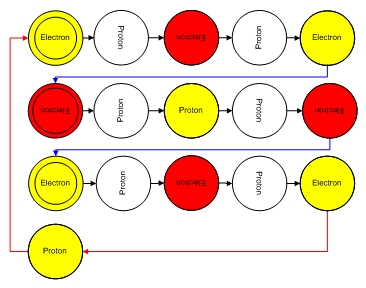# The Balls DiagramThe Balls Diagram

As you know, didactics requires some memorable associations and I thougtht about this one while listenig to Chris Matthews' Hardball. Valentine's Day was also in my mind...:)

So let's learn some physics up, Fellas.:)

The Balls Diagram has been shown for the initial particles of the Hyperon Family and even though they should be self-evident, I thought that it would be worthwhile to spend a blog on it...:)

Let's take the Coherence Diagram for the Pion Minus for simplicity and its corresponding Balls Diagram.

Pion Minus

Below is the amazing Pion Minus chord which is composed of four chords or 16 notes.As you should know by now, the Coherence Diagram showcases a bunch of sequential transitions among different 4D stationary deformational states. As you can see I recasted the states in terms 2/3 and 1/3 and their negative values. The meaning of these states depends not only upon their displacement axis lenght amplitude but also in the phase of coherence. This means that event though the lowest state of the left well (0,-2/3,-1/3) is being associated with the electron state (3D projection of the 4D displacement volume proportional to the 3D mass of an electron), it can also represent a positron (0,2/3,1/3).

Notice that the Zero is in bold, meaning that the axis perpendicular to the Radial Direction R and the axis X is being defined as the axis of spinning. It was choosen like that to provide the smallest 4D volume of Rotation on the Neutron Decay equation. The reasoning is slightly convoluted, but that is how complex assignments are made.

Neutron -> Proton + Electron + Anti-Neutrino
(2/3,-1/3,-1/3) -> (2/3, 1/3,0) + (0,-2/3,-1/3)
-> (2/3, 2/3,-1/3) + (0,-2/3,-1/3) + (0,-1/3,1/3)

We presented the Electron with the smallest 4D volume which would imply that the axis of revolution will be perpendicular to the smallest axis (x-axis has zero displacement axis length). Notice that the electron-proton dilator is the fundamental coherence, thus any spinning will have to keep its axis or spinning. The final assignment with respect to which spinning axis one should use is still under discussion in my mind.

An alternative assignment is:
Neutron -> Proton + Electron + Anti-Neutrino
(2/3,-1/3,-1/3) -> (2/3, 1/3,0) + (0,-2/3,-1/3)
-> (2/3, 2/3,-1/3) + (0,-2/3,-1/3) + (0,-1/3,1/3)

This assignment uses the smallest axis of the Proton instead. In fact, this seems to be a better assigment since is also makes the lowest volume for the Neutron. Although the electon would be spinning around a larger axis, the volume associated with the other two axes is zero or thereabouts. This is easy to see if you forget the 4D ellipsoid of revolution for a second and think about the particle as a parallellepiped with three axis. In the case of the electron one of them has zero lenght, thus the area associated with it would be zero, thus spinning it wouldn't give you that much volume.

This is a better assignment after all, thus disregard some bolding in the wrong axis until I correct this mistake... As you know, one has to have Balls to accepts one's mistakes...:)

Courage will also suffice....:)

Notice that the double potential well is more complex than I let you know. The choice of which axis you are spinning breaks down the spinning degeneracy of the problem further. I will overlook this now, otherwise I am sure you will blow a gasket...:)

Notice that an alternative view of the Neutron Coherence or transition state is a dilator quadrupole.

This is consistent with all the other also having the smallest 4D Rotational Volume and thus 3D masses. That is why I wrote the equation as such. Different axis of rotation would result in a different mass and be recognized as a different particle, made up by a set of different quarks by our creative scientists...:)

Now that we understand a little more about the Coherence Diagram, one can ask questions about why the two Electron states are not degenerate. They differ just by a spatial rotation (y into z and z into y)...:) The reason is simple even though state is isotropic or quasi-isotropic, there is a difference in pointing a 4D spatial deformation in one direction or another. To change the direction of deformation one has to do work, dynamically. That dynamic effort (as opposed to adiabatic work) is responsible for the dynamical symmetry breakdown. It is not a energy difference that makes this states different, it is the fact that the dilator has to physically redirect itself and that takes time. So the states (0,-2/3,-1/3) don't differ in energy (4D volume) but they are distinct.

The same is valid for the muon and tau neutrinos associated with the proton side. I did not refer to them yet since I did not mention any decaying particle that emits muon and tau neutrinos. I will do so later.

This dynamic state change takes time and that is happening while the dilator is rotating (spinning) on the 4D space.

This complex coherence or chord can also be shown as a Balls Diagram:Let analyse events Ball by Ball or note by note, if you so wish...:) Let's start with the electron chord. Starting from the top as a musician would say..>:)

The Pion chord is composed of two electron chords, one positron chord, one eletron-positron transmutation note, one positron-electron transmutation note, one eletron-proton transmutation note and finally a proton-electron transmutation note.

If you look close enough at the Coherence Diagram, you will be able to see which states are involved with each of these transmutation notes.

Let's start with the Electron-Positron transmutation note. This note corresponds to two arrows in our coherence diagram. The first arrow starts at the (0,-2/3,-1/3) and ends on (0,-1/3,-2/3). The second arrow starts at (0,-1/3,-2/3) and ends back on (0,-2/3,-1/3). This transition has the physical meaning of a spatial rotation in 3D and has angular momentum associate with it.

The time it takes to make this 3D spatial rotation is such that the tunneling goes from contraction to expansion, that is, the displacement volume goes from (0,-2/3,-1/3) to (0,2/3,1/3), or the state goes from electron to positron and it is also the time or thereabouts it takes to do a 180 degrees spinning 4D rotation. This has to be the case otherwise one wouldn't see that positive charge component of the chord. The meaning of thereabouts is because the 180 degrees 4D spinning cycle is not the same as the 180 degrees 3D rotational cycle. This causes the positron to be crocked...:) As you know, this is a local deformation of the fabric of space and corresponds to a virtual velocity. These virtual velocities are the spring that stores the nuclear energy. It is not necessary fields, gluons and any other sticky crap...:)

One should eventually define the exact shape of this potential well and the "Schrodinger" equation for the dilator. I will do that in the appropriate time and this is quite a simple task within this logical framework.

Now let's set our sights back onto the Balls Diagram. The first four balls are the four notes of the electron chord. The blue line between the last electron ball and the first positron ball is blue for a reason....:) It is blue because it corresponds to a transmutation note and it is equal to the positron-electron transmutation note. Symmetry tells me that they are identical, that is, it takes the same time to make each 90 degrees spatial rotation.

I should mention that upside particles are normal particles while upside down are anti-particles (dilators with a 180 degrees dephase). This means that one electron contracts 4D space while a positron expands 4D space.

There is an important feature that people might be missing. Sidewise particles are not seen on the 3D Universe. These states have little aberration (height) along the radial direction, so when they spin sidewise, they have no interaction with the 3D Universe.

Of course, eventually the energy stored in those motions survive in the form of flying products. The energy is stored in the Fabric of Space twisting, the transmutation chords (an electron-positron plus a positron-electron transmutation notes together make up a neutrino chord).

The electron-proton plus proton-electron-proton notes unravel together with the extra positron-electron pair such as to deform space enough to propel the remaining particle at their emission speeds.

That is the meaning of the Balls Diagram and a more detailed explanation on the nature of matter.

If you want to understand someting deeper, read my old blog on the Meaning of Material Existence.

Feynman created his Feynman diagrams, Einstein left us with Einstein's equations, Newton left Newton's Laws... I am starting to worry about my legacy...:)

Good forbid it from being my .... :)

Cheers,

MP

Currently unrated

### New Comment

required

required (not published)

optional

required